Tag: Dedekind

Here the details of the iguanodon series. Start with the Farey sequence $F(n)$of order n which is the sequence of completely reduced fractions between 0 and 1 which, when in lowest terms, have denominators less than or equal to n, arranged in order of increasing size. Here are the first eight Fareys

F(1) = {0⁄1, 1⁄1}
F(2) = {0⁄1, 1⁄2, 1⁄1}
F(3) = {0⁄1, 1⁄3, 1⁄2, 2⁄3, 1⁄1}
F(4) = {0⁄1, 1⁄4, 1⁄3, 1⁄2, 2⁄3, 3⁄4, 1⁄1}
F(5) = {0⁄1, 1⁄5, 1⁄4, 1⁄3, 2⁄5, 1⁄2, 3⁄5, 2⁄3, 3⁄4, 4⁄5, 1⁄1}
F(6) = {0⁄1, 1⁄6, 1⁄5, 1⁄4, 1⁄3, 2⁄5, 1⁄2, 3⁄5, 2⁄3, 3⁄4, 4⁄5, 5⁄6, 1⁄1}
F(7) = {0⁄1, 1⁄7, 1⁄6, 1⁄5, 1⁄4, 2⁄7, 1⁄3, 2⁄5, 3⁄7, 1⁄2, 4⁄7, 3⁄5, 2⁄3, 5⁄7, 3⁄4, 4⁄5, 5⁄6, 6⁄7, 1⁄1}
F(8) = {0⁄1, 1⁄8, 1⁄7, 1⁄6, 1⁄5, 1⁄4, 2⁄7, 1⁄3, 3⁄8, 2⁄5, 3⁄7, 1⁄2, 4⁄7, 3⁄5, 5⁄8, 2⁄3, 5⁄7, 3⁄4, 4⁄5, 5⁄6, 6⁄7, 7⁄8, 1⁄1}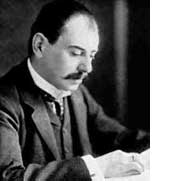Farey sequences have plenty of mysterious properties. For example, in 1924 J. Franel and Edmund Landau proved that an asymptotic density result about Farey sequences is equivalent to the Riemann hypothesis.
More precisely, let a(n) be the number of terms in the Farey sequence F(n) (that is, a(1)=2,a(2)=3,…,a(8)=23 etc. This is sequence A005728 in the online integer sequences catalog).
Let $F(n)_j$ denote the j-th term in F(n), then the following conjecture is equivalent to the Riemann hypothesis

For every $\epsilon > 0$ there is a constant C depending on $\epsilon$ such that

$\sum_{j=1}^{a(n)} | F(n)_j – \frac{j}{a(n)} | < C n^{\frac{1}{2}+\epsilon}$

when n goes to infinity. Anyway, let us continue our construction. Farey sequences are clearly symmetric around 1/2 so let us just take half of them, so we jump to 1 when we have reached 1/2. Let us extend this halved Farey on both sides with $\infty$ and call it the modified Farey sequence f(n). For example,

$f(3) = {~\infty,0,\frac{1}{3},\frac{1}{2},1,\infty }$

Now consider the Farey code in which we identify the two sides connected to $\infty$ and mark two consecutive Farey numbers as

$$\xymatrix{f(n)_i \ar@{-}[r]_{\bullet} & f(n)_{i+1}}$$

That is, the Farey code associated to the modified sequence f(3) is

$$\xymatrix{\infty \ar@{-}[r]_{1} & 0 \ar@{-}[r]_{\bullet} & \frac{1}{3} \ar@{-}[r]_{\bullet} & \frac{1}{2} \ar@{-}[r]_{\bullet} & 1 \ar@{-}[r]_{1} & \infty}$$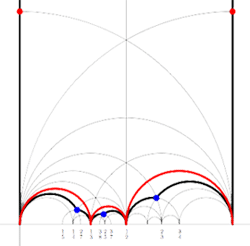Recall from earlier that to a Farey-code we can associate a special polygon by first taking the hyperbolic convex hull of all the terms in the sequence (the region bounded by the vertical lines and the bottom red circles in the picture on the left) and adding to it for each odd interval $$\xymatrix{f(n)_i \ar@{-}[r]_{\bullet} & f(n)_{i+1}}$$ the triangle just outside the convex hull consisting of two odd edges in the Dedekind tessellation (then we obtain the region bounded by the black geodesics for the sequence f(3)).

Next, we can associate to this special polygon a cuboid tree diagram by considering all even and odd vertices on the boundary (which are tinted red, respectively blue) together with all odd vertices in the interior of the special polygon. These are indicated in the left picture below. If we connect these vertices with the geodesics in the polygon we get a cuboid tree diagram. The obtained cuboid tree diagram is depicted on the right below.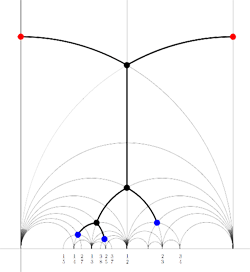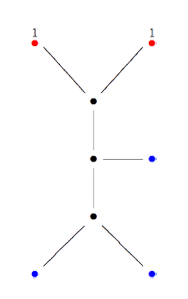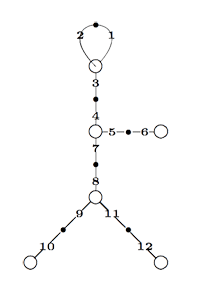Finally, identifying the red points (as they lie on geodesics connected to $\infty$ which are identified in the Farey code), adding even points on the remaining geodesics and numbering the obtained half-lines we obtain the dessin d’enfant given on the left hand side. To such a dessin we can associate its monodromy group which is a permutation group on the half-lines generated by an order two element indicating which half-lines make up a line and an order three element indicating which half-lines one encounters by walking counter-clockwise around a three-valent vertex. For the dessin on the left the group is therefore the subgroup of $S_{12}$ generated by the elements

$\alpha = (1,2)(3,4)(5,6)(7,8)(9,10)(11,12)$

$\beta = (1,2,3)(4,5,7)(8,9,11)$

and a verification with GAP tells us that this group is the sporadic Mathieu group $M_{12}$. This concludes the description of the second member of the Iguanodon series. If you like to check that the first 8 iguanodons are indeed the simple groups

$L_2(7), M_{12}, A_{16}, M_{24}, A_{28}, A_{40}, A_{48}, A_{60}, \ldots$

the following dissection of the Iguanodon may prove useful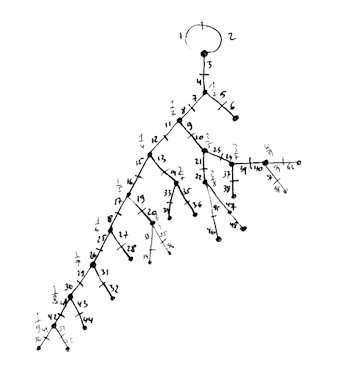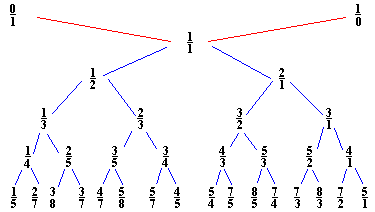John Farey (1766-1826) was a geologist of sorts. Eyles, quoted on the math-biographies site described his geological work as

“As a geologist Farey is entitled to respect for the work which he carried out himself, although it has scarcely been noticed in the standard histories of geology.”

That we still remember his name after 200 years is due to a short letter he wrote in 1816 to the editor of the Philosophical Magazine

“On a curious Property of vulgar Fractions.
By Mr. J. Farey, Sen. To Mr. Tilloch

Sir. – On examining lately, some very curious and elaborate Tables of “Complete decimal Quotients,” calculated by Henry Goodwyn, Esq. of Blackheath, of which he has printed a copious specimen, for private circulation among curious and practical calculators, preparatory to the printing of the whole of these useful Tables, if sufficient encouragement, either public or individual, should appear to warrant such a step: I was fortunate while so doing, to deduce from them the following general property; viz.

If all the possible vulgar fractions of different values, whose greatest denominator (when in their lowest terms) does not exceed any given number, be arranged in the order of their values, or quotients; then if both the numerator and the denominator of any fraction therein, be added to the numerator and the denominator, respectively, of the fraction next but one to it (on either side), the sums will give the fraction next to it; although, perhaps, not in its lowest terms.

For example, if 5 be the greatest denominator given; then are all the possible fractions, when arranged, 1/5, 1/4, 1/3, 2/5, 1/2, 3/5, 2/3, 3/4, and 4/5; taking 1/3, as the given fraction, we have (1+1)/(5+3) = 2/8 = 1/4 the next smaller fraction than 1/3; or (1+1)/(3+2) = 2/5, the next larger fraction to 1/3. Again, if 99 be the largest denominator, then, in a part of the arranged Table, we should have 15/52, 28/97, 13/45, 24/83, 11/38, &c.; and if the third of these fractions be given, we have (15+13)/(52+45) = 28/97 the second: or (13+11)/(45+38) = 24/83 the fourth of them: and so in all the other cases.

I am not acquainted, whether this curious property of vulgar fractions has been before pointed out?; or whether it may admit of any easy or general demonstration?; which are points on which I should be glad to learn the sentiments of some of your mathematical readers; and am

J. Farey. Howland-street.”

So, if we interpolate “childish addition of fractions” $\frac{a}{b} \oplus \frac{c}{d} = \frac{a+c}{b+d}$ and start with the numbers $0 = \frac{0}{1}$ and $\infty = \frac{1}{0}$ we get the binary Farey-tree above. For a fixed natural number n, if we stop the interpolation whenever the denominator of the fraction would become larger than n and order the obtained fractions (smaller or equal to one) we get the Farey sequence F(n). For example, if n=3 we start with the sequence $\frac{0}{1},\frac{1}{1}$. The next step we get $\frac{0}{1},\frac{1}{2},\frac{1}{1}$ and the next step gives

$\frac{0}{1},\frac{1}{3},\frac{1}{2},\frac{2}{3},\frac{1}{1}$

and as all the denomnators of childish addition on two consecutive fractions will be larger than 3, the above sequence is F(3). A remarkable feature of the series F(n) is that if $\frac{a}{b}$ and $\frac{c}{d}$ are consecutive terms in F(n), then

$det \begin{bmatrix} a & c \\\ b & d \end{bmatrix} = -1$

and so these two fractions are the endpoints of an even geodesic in the Dedekind tessellation.

A generalized Farey series is an ordered collection of fractions $\infty,x_0,x_1,\cdots,x_n,\infty$ such that $x_0$ and $x_n$ are integers and some $x_i=0$. Moreover, writing $x_i = \frac{a_i}{b_i}$ we have that

$det \begin{bmatrix} a_i & a_{i+1} \\\ b_i & b_{i+1} \end{bmatrix} = -1$

A Farey code is a generalized Farey sequence consisting of all the vertices of a special polygon that lie in $\mathbb{R} \cup \{ \infty \}$ together with side-pairing information. If two consecutive terms are such that the complete geodesic between $x_i$ and $x_{i+1}$ consists of two sides of the polygon which are paired we denote this fact by
$$\xymatrix{x_i \ar@{-}[r]_{\circ} & x_{i+1}}$$. If they are the endpoints of two odd sides of the polygon which are paired we denote this by $$\xymatrix{x_i \ar@{-}[r]_{\bullet} & x_{i+1}}$$. Finally, if they are the endpoints of a free side which is paired to another free side determined by $x_j$ and $x_{j+1}$ we denote this fact by marking both edges $$\xymatrix{x_i \ar@{-}[r]_{k} & x_{i+1}}$$ and $$\xymatrix{x_j \ar@{-}[r]_{k} & x_{j+1}}$$ with the same number.For example, for the M(12) special polygon on the left (bounded by the thick black geodesics), the only vertices in $\mathbb{R} \cup \{ \infty \}$ are $\infty,0,\frac{1}{3},\frac{1}{2},1$. The two vertical lines are free sides and are paired, whereas all other sides of the polygon are odd. Therefore the Farey-code for this Mathieu polygon is

$$\xymatrix{\infty \ar@{-}[r]_{1} & 0 \ar@{-}[r]_{\bullet} & \frac{1}{3} \ar@{-}[r]_{\bullet} & \frac{1}{2} \ar@{-}[r]_{\bullet} & 1 \ar@{-}[r]_{1} & \infty}$$

Conversely, to a Farey-code we can associate a special polygon by first taking the hyperbolic convex hull of all the terms in the sequence (the region bounded by the vertical lines and the bottom red circles in the picture on the left) and adding to it for each odd interval $$\xymatrix{x_i \ar@{-}[r]_{\bullet} & x_{i+1}}$$ the triangle just outside the convex hull consisting of two odd edges in the Dedekind tessellation (then we obtain the region bounded by the black geodesics). Again, the side-pairing of the obained special polygon can be obtained from that of the Farey-code.

This correspondence gives a natural one-to-one correspondence special polygons < ---> Farey-codes . _Later_ we will see how the Farey-code determines the group structure of the corresponding finite index subgroup of the modular group $\Gamma = PSL_2(\mathbb{Z})$.

Reference

Ravi S. Kulkarni, “An arithmetic-geometric method in the study of the subgroups of the modular group”, Amer. J. Math 113 (1991) 1053-1133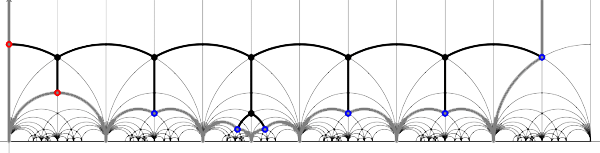Today we will link modular quilts (via their associated cuboid tree diagrams) to special hyperbolic polygons. The above drawing gives the hyperbolic polygon (the gray boundary) associated to the M(24) tree diagram (the black interior graph). In general, the correspondence goes as follows.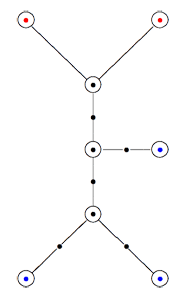Recall that a cuboid tree diagram is a tree such that all internal vertices are 3-valent and have a specified ordering on the incident edges (given by walking counterclockwise around the vertex) and such that all leaf-vertices are tinted blue or red, the latter ones are paired via an involution (indicated by giving paired red vertices the same label). Introduce a new 2-valent vertex on all edges joining two internal vertices or a blue vertex to an internal vertex. So, the picture on the right corresponds to the tree diagram on the left. Equip this extended tree with a metric such that every edge has length equal to an f-edge in the Dedekind tessellation. Fix an edge having a red vertex and develop this isometrically onto the f-edge connecting $i$ to $\rho$ in the tessellation. Then, the extended tree develops uniquely along the f-edges of the tessellation and such that the circled black and blue vertices correspond to odd vertices, the circled red and added uncircled vertices correspond to even vertices in the tessellation. Starting from the above tree (and choosing the upper-left edge to start the embedding), we obtain the picture on the left (we have removed the added 2-valent vertices)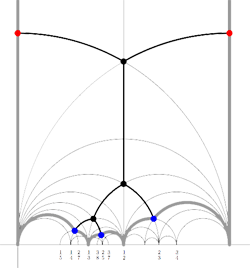We will now associate a special hyperbolic polygon to this tree. At a red vertex take the even line going through the vertex. If under the involution the red vertex is send to itself, the even edges will be paired. Otherwise, the line is a free side and will be paired to the free side containing the red vertex corresponding under the involution. At a blue vertex, take the two odd edges making an angle of $\frac{\pi}{3}$ with the tree-edge containing the blue vertex. These odd edges will be paired. If we do this procedure for all blue and red vertices, we obtain a special polygon (see the picture on the right, the two vertical lines are paired).
Conversely, suppose we start with a special polygon such as the one on the left below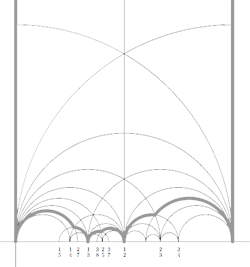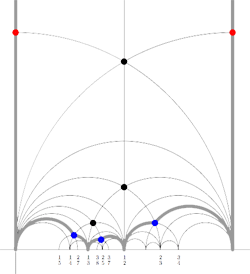and consider all even and odd vertices on the boundary (which are tinted red, respectively blue) together with all odd vertices in the interior of the special polygon. These are indicated in the picture on the right above. If we connect these vertices with the geodesics in the polygon we get a cuboid tree diagram. This correspondence special polygons —>> tree diagrams is finite to one as we have made a choice in the starting red vertex and edge. If we would have taken the other edge containing a red vertex we would end up with the following special polygon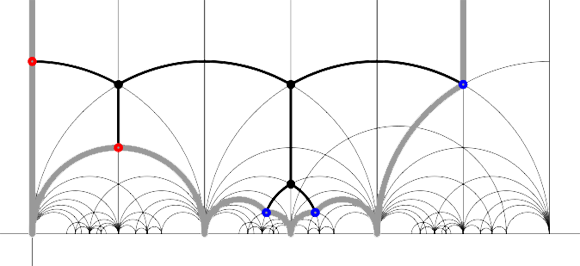It is no accident that these two special polygons consist of exactly 24 triangles of the Dedekind tessellation as they correspond to the index 12 subgroup of the modular group $\Gamma$ determining the 12-dimensional permutation representation of the Mathieu group $M_{12}$. Similarly, the top drawing has 48 hyperbolic triangles and corresponds to the 24-dimensional permutation representation of $M_{24}$. Another time we will make the connection with Farey series which will allow us to give free generators of finite index subgroups.

Reference

Ravi S. Kulkarni, “An arithmetic-geometric method in the study of the subgroups of the modular group”, Amer. J. Math. 113 (1991) 1053-1133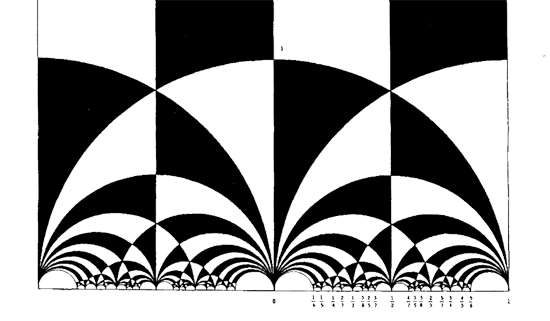In 1877, Richard Dedekind discovered one of the most famous pictures in mathematics : the black&white tessellation of the upper half-plane in hyperbolic triangles. Recall that the group $SL_2(\mathbb{Z})$ of all invertible 2×2 integer matrices with determinant $1$ acts on the upper halfplane via

The
natural habitat of this lesson is a bit further down the course, but it
was called into existence by a comment/question by
Kea

I don’t yet quite see where the nc
manifolds are, but I guess that’s coming.

As
I’m enjoying telling about all sorts of sources of finite dimensional
representations of $SL_2(\mathbb{Z})$ (and will carry on doing so for
some time), more people may begin to wonder where I’m heading. For this
reason I’ll do a couple of very elementary posts on simple examples of
noncommutative manifolds.

I realize it is ‘bon ton’ these days
to say that noncommutative manifolds are virtual objects associated to
noncommutative algebras and that the calculation of certain invariants
of these algebras gives insight into the topology and/or geometry of
these non-existent spaces. My own attitude to noncommutative geometry is
different : to me, noncommutative manifolds are genuine sets of points
equipped with a topology and other structures which I can use as a
mnemotechnic device to solve the problem of interest to me which is the
classification of all finite dimensional representations of a smooth
noncommutative algebra.

Hence, when I speak of the
‘noncommutative manifold of $SL_2(\mathbb{Z})$’ Im after an object
containing enough information to allow me (at least in principle) to
classify the isomorphism classes of all finite dimensional
$SL_2(\mathbb{Z})$-representations. The whole point of this course is
to show that such an object exists and that we can make explicit
an elementary question :

Riemann surfaces are examples of
noncommutative manifolds, so what is the noncommutative picture of
them?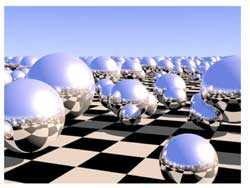I’ve browsed the Google-pictures a bit and a picture
coming close to my mental image of the noncommutative manifold of a
Riemann surface locally looks like the picture on the left. Here, the checkerboard-surface is part of the Riemann surface
and the extra structure consists in putting in each point of the Riemann
surface a sphere, reflecting the local structure of the Riemann surface
near the point. In fact, my picture is slightly different : I want to
draw a loop in each point of the Riemann surface, but Ill explain why
the two pictures are equivalent and why they present a solution to the
problem of classifying all finite dimensional representations of the
Riemann surface. After all why do we draw and study Riemann
surfaces? Because we are interested in the solutions to equations. For
example, the points of the _Kleinian quartic Riemann
surface_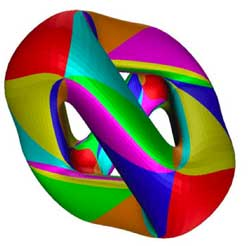give us all solutions tex \in
\mathbb{C}^3 $to the equation$X^3Y+Y^3Z+Z^3X=0 $. If (a,b,c) is such a solution, then so are all scalar multiples$(\lambda a,\lambda
b,\lambda c) $so we may as well assume that the Z$coordinate is equal
to 1 and are then interested in finding the solutions tex \in
\mathbb{C}^2 $to the equation$X^3Y+Y^3+X=0 $which gives us an affine patch of the Kleinian quartic (in fact, these solutions give us all points except for two, corresponding to the _points at infinity_ needed to make the picture compact so that we can hold it in our hand and look at it from all sides. These points at infinity correspond to the trivial solutions (1,0,0) and (0,1,0)). What is the connection between points on this Riemann surface and representations? Well, if (a,b) is a solution to the equation$X^3Y+Y^3+X=0 $, then we have a _one-dimensional representation_ of the affine _coordinate ring_$\mathbb{C}[X,Y]/(X^3Y+Y^3+X) $, that is, an algebra morphism$\mathbb{C}[X,Y]/(X^3Y+Y^3+X) \rightarrow \mathbb{C} $defined by sending X to a and Y to b. Conversely, any such one-dimensonal representation gives us a solution (look at the images of X and Y and these will be the coordinates of a solution). Thus, commutative algebraic geometry of smooth curves (that is Riemann surfaces if you look at the ‘real’ picture) can be seen as the study of one-dimensional representations of their smooth coordinate algebras. In other words, the classical Riemann surface gives us already the classifcation of all one-dimensional representations, so now we are after the ‘other ones’. In noncommtative algebra it is not natural to restrict attention to algebra maps to$\mathbb{C} $, at least we would also like to include algebra maps to$n \times n $matrices$M_n(\mathbb{C}) $. An n-dimensional representation of the coordinate algebra of the Klein quartic is an algebra map$\mathbb{C}[X,Y]/(X^3Y+Y^3+X) \rightarrow M_n(\mathbb{C}) $That is, we want to find all pairs of$n \times n $matrices A and B satisfying the following matrix-identities$A.B=B.A $and$A^3.B+B^3+A=0_n $The first equation tells us that the two matrices must commute (because we took commuting variables X and Y) and the second equation really is a set of$n^2 $-equations in the matrix-entries of A and B. There is a sneaky way to get lots of such matrix-couples from a given solution (A,B), namely by _simultaneous conjugation_. That is, if$C \in GL_n(\mathbb{C}) $is any invertible$n \times n $matrix, then also the matrix-couple$~(C^{-1}.A.C,C^{-1}.B.C) $satisfies all the required equations (write the equations out and notice that middle terms of the form$C.C^{-1} $cancel out and check that one then obtains the matrix-identities$C^{-1} A B C = C^{-1} BA C $and$C^{-1}(A^3B+B^3+A)C = 0_n $which are satisfied because (A,B) was supposed to be a solution). We then say that these two n-dimensional representations are _isomorphic_ and naturally we are only interested in classifying the isomorphism classes of all representations. Using classical commutative algebra theory of Dedekind domains (such as the coordinate ring$\mathbb{C}[X,Y]/(X^3Y+Y^3+X) $) allows us to give a complete solution to this problem. It says that any n-dimensional representation is determined up to isomorphism by the following geometric/combinatorial data • a finite set of points$P_1,P_2,\dots,P_k $on the Riemann surface with$k \leq n $. • a set of positive integers$a_1,a_2,\dots,a_k $associated to these pointssatisfying$a_1+a_2+\dots_a_k=n $. • for each$a_i $a partition of$a_i $(that is, a decreasing sequence of numbers with total sum$a_i $). To encode this classification I’ll use the mental picture of associating to every point of the Klein quartic a small loop.$\xymatrix{\vtx{}
\ar@(ul,ur)} $Don\’t get over-exited about this noncommutative manifold picture of the Klein quartic, I do not mean to represent something like closed strings emanating from all points of the Riemann surface or any other fanshi-wanshi interpretation. Just as Feynman-diagrams allow the initiated to calculate probabilities of certain interactions, the noncommutative manifold allows the initiated to classify finite dimensional representations. Our mental picture of the noncommutative manifold of the Klein quartic, that is : the points of the Klein quartic together with a loop in each point, will tell the initiated quite a few things, such as : The fact that there are no arrows between distict points, tells us that the classification problem splits into local problems in a finite number of points. Technically, this encodes the fact that there are no nontrivial extensions between different simples in the commutative case. This will drastically change if we enter the noncommutative world…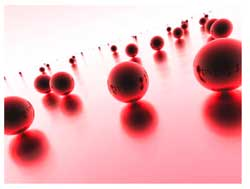The fact that there is one loop in each point, tells us that the local classification problem in that point is the same as that of classifying nilpotent matrices upto conjugation (which, by the Jordan normal form result, are classified by partitions) Moreover, the fact that there is one loop in each point tells us that the local structure of simple representations near that point (that is, the points on the Kleinian quartic lying nearby) are classified as the simple representations of the polynmial algebra$\mathbb{C}[x] $(which are the points on the complex plane, giving the picture of the Riemann sphere in each point reflecting the local neighborhood of the point on the Klein quartic) In general, the noncommutative manifold associated to a noncommutative smooth algebra will be of a similar geometric/combinatorial nature. Typically, it will consist of a geometric collection of points and arrows and loops between these points. This data will then allow us to reduce the classification problem to that of _quiver-representations_ and will allow us to give local descriptions of our noncommutative manifolds. Next time, I’ll give the details in the first noncommutative example : the skew-group algebra of a finite group of automorphisms on a Riemann surface (such as the simple group$PSL_2(\mathbb{F}_7) \$ acting on the
Klein quartic). Already in this case, some new phenomena will
appear…

ADDED : While writing this post
NetNewsWire informed me that over at Noncommutative Geometry they have a
post on a similar topic : What is a noncommutative space.